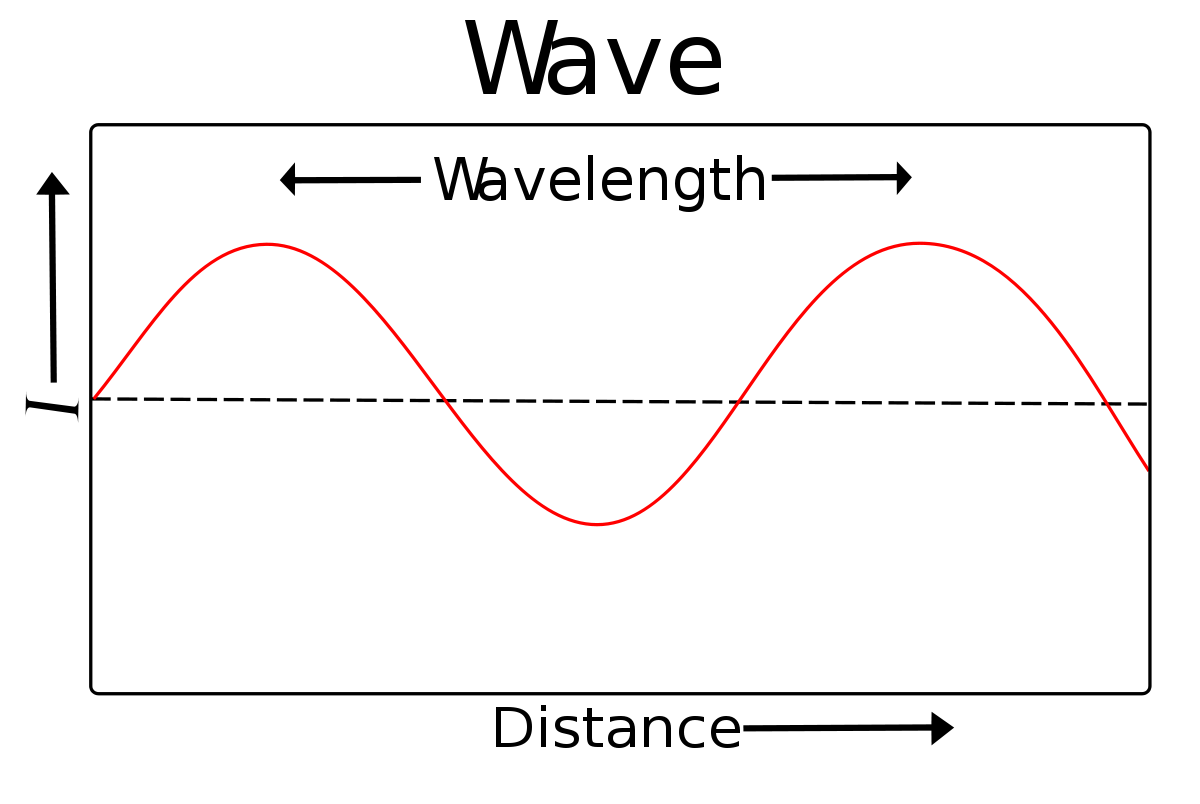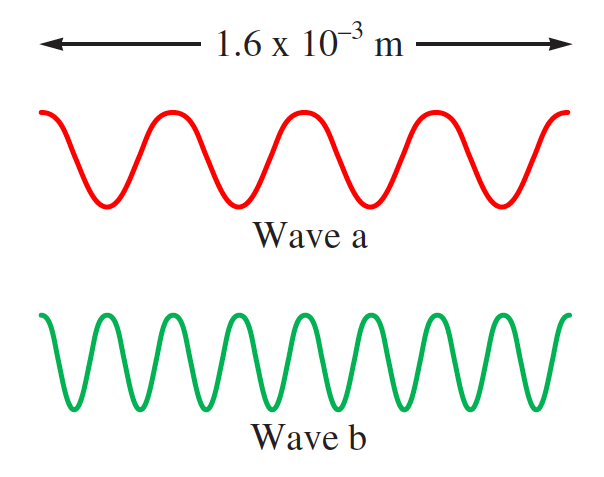# Problem: Consider the following waves representing electromagnetic radiation:Which wave has the longer wavelength? Calculate the wavelength. Which wave has the higher frequency and larger photon energy? Calculate these values. Which wave has the greater velocity? What type of electromagnetic radiation does each wave represent?

###### FREE Expert Solution

Which wave has the longer wavelength? Calculate the wavelength.

• Recall that the definition of wavelength is the measure of the length between two “peaks”• It appears that Wave A has the longer wavelength

93% (184 ratings)###### Problem Details

Consider the following waves representing electromagnetic radiation:Which wave has the longer wavelength? Calculate the wavelength. Which wave has the higher frequency and larger photon energy? Calculate these values. Which wave has the greater velocity? What type of electromagnetic radiation does each wave represent?

Frequently Asked Questions

What scientific concept do you need to know in order to solve this problem?

Our tutors have indicated that to solve this problem you will need to apply the Electromagnetic Spectrum concept. You can view video lessons to learn Electromagnetic Spectrum. Or if you need more Electromagnetic Spectrum practice, you can also practice Electromagnetic Spectrum practice problems.

What professor is this problem relevant for?

Based on our data, we think this problem is relevant for Professor Cruz's class at USF.

What textbook is this problem found in?

Our data indicates that this problem or a close variation was asked in Chemistry: An Atoms First Approach - Zumdahl Atoms 1st 2nd Edition. You can also practice Chemistry: An Atoms First Approach - Zumdahl Atoms 1st 2nd Edition practice problems.